# Nyström Approximation¶

While linear techniques operating in the primal (input) space are able to achieve good generalization capabilities in some specific application areas, one cannot in general approximate with the linear model more complex or highly nonlinear functions. We apply a Fixed-Size approach [FS2010] and Nyström approximation [WS2001] to approximate a kernel-induced feature map with some higher dimensional explicit and approximate feature vector.

We select prototype vectors (a small working sample of size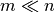) and construct, for instance an RBF kernel matrix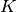with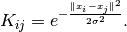By following the approach in [WS2001] an expression for the entries of the approximated feature map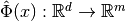, with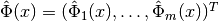is given by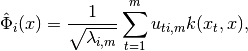where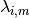and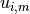denote the i-th eigenvalue and the i-th eigenvector of.

## Available API¶

AFEm(Xs, kernel, X)

Performs Automatic Feature Extraction (AFE) by Nyström method [WS2001] using a subsample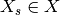. We restrict kernel <: Kernel to be a subclass of Kernel, for instance RBFKernel.

Parameters: Xs – subset which is used to construct kernel matrixkernel – kernel function, e.g. RBFKernel(), used to construct kernel matrixX – full dataset new dataset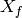derived from stacking together feature maps for every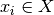entropy_subset(X, kernel, subset_size)

Performs maximization of the quadratic Rényi Entropy by the representative points selection from X which can be supplied to AFEm as Xs subset.

Parameters: X – full dataset kernel – kernel function, e.g. RBFKernel(), used to construct kernel matrixover which we compute Rényi Entropy subset_size – number of representative data points

## Available Kernel Functions¶

LinearKernel()

Defines an implementation of the Linear Kernel, i.e.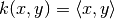.

PolynomialKernel()

Defines an implementation of the Polynomial Kernel, i.e.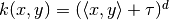.

RBFKernel()

Defines an implementation of the Radial Basis Function (RBF) Kernel, i.e.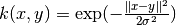.

 [WS2001] (1, 2, 3) Williams C. and Seeger M., “Using the Nyström method to speed up kernel machines”, in Proceedings of the 14th Annual Conference on Neural Information Processing (NIPS), pp. 682-688, 2001.